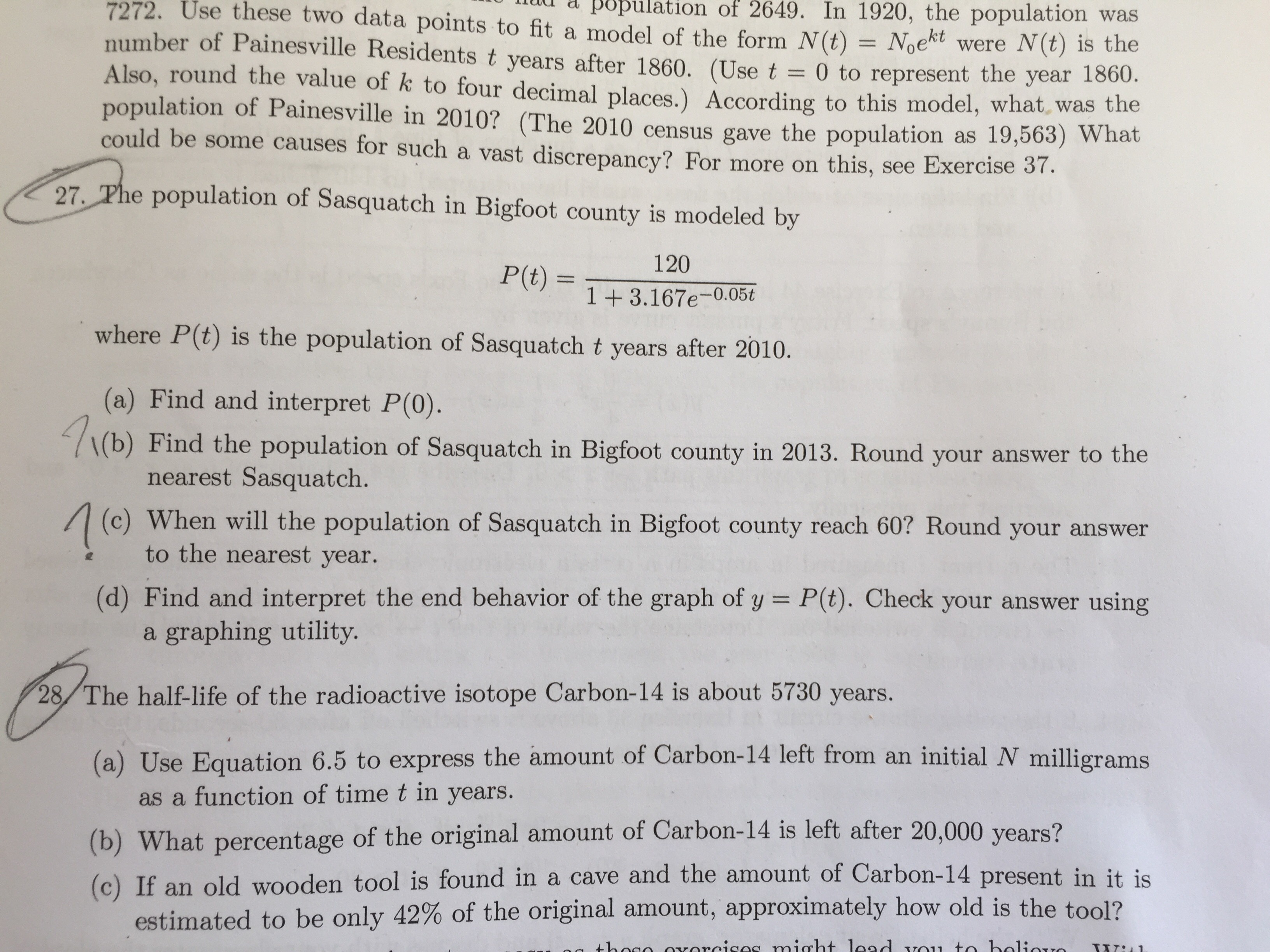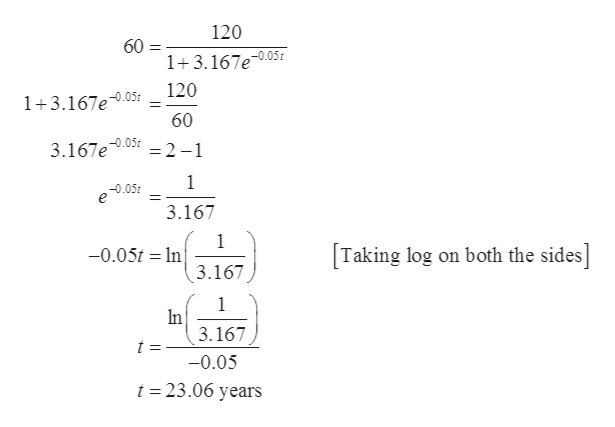# ulation of 2649. In 1920, the population was7272. Use these two data points to fit a model of the form N(t)number of Painesville Residents t years after 1860. (Use tAlso, round the value of k to four decimal places.) According to this model, what was thepopulation of Painesville in 2010? (The 2010 census gave the population as 19,563) Whatcould be some causes for such a vast discrepancy? For more on this, see Exercise 37.Noektwere N(t) is the0 torepresent the year 1860.27 The population of Sasquatch in Bigfoot county is modeled by120P(t)1+3.167e-0.05twhere P(t) is the population of Sasquatch t years after 2010.(a) Find and interpret P(0).1(b) Find the population of Sasquatch in Bigfoot county in 2013. Round your answer to thenearest Sasquatch.When will the population of Sasquatch in Bigfoot county reach 60? Round your answerto the nearest year.(d) Find and interpret the end behavior of the graph of y = P(t). Check your answer usinga graphing utility.28/The half-life of the radio active isotope Carbon-14 is about 5730 years.(a) Use Equation 6.5 to express the amount of Carbon-14 left from an initial N milligramsas a function of time t in years.(b) What percent age of the original amount of Carbon-14 is left after 20,000 years?(c) If an old wooden tool is found in a cave and the amount of Carbon-14 present in it isestimated to be only 42% of the original amount, approximately how old is the tool?

Question

Question 27 - Letter (c)help_outlineImage Transcriptioncloseulation of 2649. In 1920, the population was 7272. Use these two data points to fit a model of the form N(t) number of Painesville Residents t years after 1860. (Use t Also, round the value of k to four decimal places.) According to this model, what was the population of Painesville in 2010? (The 2010 census gave the population as 19,563) What could be some causes for such a vast discrepancy? For more on this, see Exercise 37. Noekt were N(t) is the 0 to represent the year 1860. 27 The population of Sasquatch in Bigfoot county is modeled by 120 P(t) 1+3.167e-0.05t where P(t) is the population of Sasquatch t years after 2010. (a) Find and interpret P(0). 1(b) Find the population of Sasquatch in Bigfoot county in 2013. Round your answer to the nearest Sasquatch. When will the population of Sasquatch in Bigfoot county reach 60? Round your answer to the nearest year. (d) Find and interpret the end behavior of the graph of y = P(t). Check your answer using a graphing utility. 28/The half-life of the radio active isotope Carbon-14 is about 5730 years. (a) Use Equation 6.5 to express the amount of Carbon-14 left from an initial N milligrams as a function of time t in years. (b) What percent age of the original amount of Carbon-14 is left after 20,000 years? (c) If an old wooden tool is found in a cave and the amount of Carbon-14 present in it is estimated to be only 42% of the original amount, approximately how old is the tool? fullscreen
check_circleExpert Solution
Step 1

To determine the time when the population of sasquatch reaches 60.

Step 2

Given:

The population of sasquatch t years after 2010 is modeled by the equation:

Step 3

Substitute 60 for P(t) and solve for ...help_outlineImage Transcriptionclose120 60 1+3.167e-0.05 120 1+3.167e0.05 60 =2-1 3.167e 0.05 1 3.167 1 -0.05t In Taking log on both the sides] 3.167 1 In 3.167 t = -0.05 t 23.06 years fullscreen

### Want to see the full answer?

See Solution

#### Want to see this answer and more?

Solutions are written by subject experts who are available 24/7. Questions are typically answered within 1 hour*

See Solution
*Response times may vary by subject and question
Tagged in

### Algebra